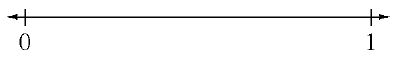### Home > CC1 > Chapter Ch6 > Lesson 6.1.1 > Problem6-13

6-13.

Copy the number line and label the following numbers at their approximate place on the number line. Homework Help ✎1. $\frac { 14 } { 28 }$

1. $0.75$

1. $\frac { 1 } { 3 }$

1. $\frac { 4 } { 7 }$

$\text{Can you decide if each portion is greater than or less than }0.5\ (\text{or }\frac{1}{2})?$

This can help you graph each portion approximately.